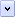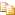Microsoft JScript provides eleven intrinsic (or "built-in") objects. They are the Array, Boolean, Date, Function, Global, Math, Number, Object, RegExp, Error, and String objects. Each of the intrinsic objects has associated methods and properties that are described in detail in the language reference. Certain objects are also described in this section.

# Array Object

The subscripts of an array can be thought of as properties of an object, are referred to by their numeric index. Note that named properties added to an Array cannot be indexed by number; they are separate from the array elements.

To create a new array, use the new operator and the Array() constructor, as in the following example.Copy Code
```var theMonths = new Array(12);
theMonths = "Jan";
theMonths = "Feb";
theMonths = "Mar";
theMonths = "Apr";
theMonths = "May";
theMonths = "Jun";
theMonths = "Jul";
theMonths = "Aug";
theMonths = "Sep";
theMonths = "Oct";
theMonths = "Nov";
theMonths = "Dec";```

When you create an array using the Array keyword, JScript includes a length property, which records the number of entries. If you do not specify a number, the length is set to 0, and the array has no entries. If you specify a number, the length is set to that number. If you specify more than one parameter, the parameters are used as entries in the array. In addition, the number of parameters is assigned to the length property, as in the following example, which is equivalent to the preceding example.Copy Code
```var theMonths = new Array("Jan", "Feb", "Mar", "Apr", "May", "Jun",
"Jul", "Aug", "Sep", "Oct", "Nov", "Dec");```

JScript automatically changes the value of length when you add elements to an array that you created with the Array keyword. Array indices in JScript always start at 0, not 1, so the length property is always one greater than the largest index in the array.

# String Object

In JScript, you can treat strings (and numbers) as if they were objects. The string Object has certain built-in methods, which you can use with your strings. One of these is the substring Method, which returns part of the string. It takes two numbers as its arguments.Copy Code
```aString = "0123456789";
var aChunk = aString.substring(4, 7);  // Sets aChunk to "456".
var aNotherChunk = aString.substring(7, 4);  // Sets aNotherChunk to "456".
// Using the preceding Array creation example:
firstLetter = theMonths.substring(0,1);  // Sets the firstLetter variable to "J".```

Another property of the String object is the length property. This property contains the number of characters in the string (0 for an empty string). This a numeric value, and can be used directly in calculations.Copy Code
`var howLong = "Hello World".length  // Sets the howLong variable to 11.`

# Math Object

The Math object has a number of predefined properties and methods. The properties are specific numbers. One of these specific numbers is the value of pi (approximately 3.14159...). This is the Math.PI property, shown in the following example.Copy Code
```// A radius variable is declared and assigned a numeric value.
var circleArea = Math.PI * radius * radius;  // Note capitalization of Math and PI.```

One of the built-in methods of the Math object is the exponentiation method, or pow, which raises a number to a specified power. The following example uses both pi and exponentiation.Copy Code
```// This formula calculates the volume of a sphere with the given radius.

# Date Object

The Date object can be used to represent arbitrary dates and times, to get the current system date, and to calculate differences between dates. It has several properties and methods, all predefined. In general, the Date object provides the day of the week; the month, day, and year; and the time in hours, minutes, and seconds. This information is based on the number of milliseconds since January 1, 1970, 00:00:00.000 GMT, which is Greenwich Mean Time (the preferred term is UTC, or "Universal Coordinated Time," which refers to signals issued by the World Time Standard). JScript can handle dates that are in the approximate range 250,000 B.C. to 255,000 A.D.

To create a new Date object, use the new operator. The following example calculates, for the current year, the number of days that have passed and the number of days that are left.Copy Code
```/*
This example uses the array of month names defined previously.
The first statement assigns today's date, in "Day Month Date 00:00:00 Year"
format, to the thisIsToday variable.
*/
var thisIsToday = new Date();

var toDay = new Date();  // Capture today's date.

// Extract the year, the month, and the day.
var thisYear = toDay.getFullYear();
var thisMonth = theMonths[toDay.getMonth()];
var thisDay = thisMonth  + " " + toDay.getDate() + ", " + thisYear;```

# Number Object

In addition to the special numeric properties (PI, for example) that are available in the Math object, several other properties are available in Microsoft JScript through the Number object.

Property Description

MAX_VALUE

Largest possible number, about 1.79E+308; can be positive or negative. (Value varies slightly from system to system.)

MIN_VALUE

Smallest possible number, about 2.22E-308; can be positive or negative. (Value varies slightly from system to system.)

NaN

Special nonnumeric value, "not a number."

POSITIVE_INFINITY

Any positive value larger than the largest positive number (Number.MAX_VALUE) is automatically converted to this value; represented as infinity.

NEGATIVE_INFINITY

Any value more negative than the largest negative number (-Number.MAX_VALUE) is automatically converted to this value; represented as -infinity.

Number.NaN is a special property that is defined as "not a number." Division by zero, for example, returns NaN. An attempt to parse a string that cannot be parsed as a number also returns Number.NaN. NaN compares unequal to any number and to itself. To test for a NaN result, do not compare against Number.NaN; use the isNaN() function instead.

Home